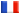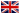# Séminaire de Cryptographie

## Françoise Levy-dit-Vehel### Algebraic Analysis of MinRank

We here investigate the hardness of one of the most relevant problems in multivariate cryptography, namely MinRank: given non-negative intgers n,k,r, and matrices M_0,...,M_k, of size n with entries in F_q, decide whether there exists an F_q-linear combination of those matrices which has rank less than or equal to r. Our starting point is the Kipnis-Shamir modeling of the problem. We first prove new properties satisfed by this modeling. Then, we propose a practical resolution of it - based on a Groebner basis approach - that permits us to efficiently solve two challenges proposed by Courtois for his zero-knowledge authentication scheme, built upon MinRank. Next we turn to the theoretical complexity of the problem: we exhibit a multi-homogeneous structure of the algebraic system modeling the probem, that yields a theoretical bound on its hardness, reflecting the practical behaviour of our approach. Our main result is that, when the size of the matrices involved minus the target rank is constant, we can solve MinRank in polynomial time.

This is a joint work with Jean-Charles Faugères and Ludovic Perret.